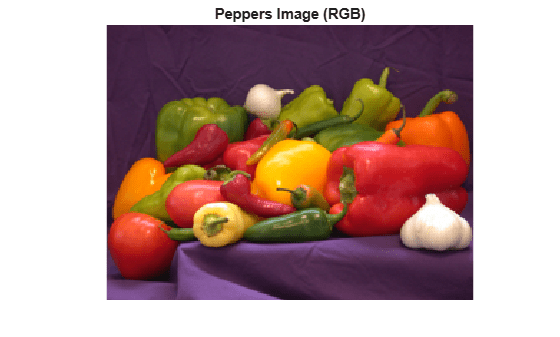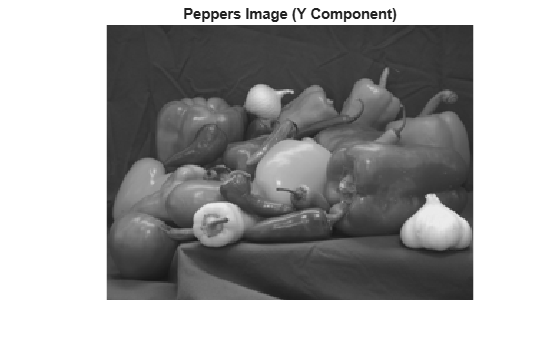## Syntax

``imageData = read(t)``
``[Y,Cb,Cr] = read(t)``

## Description

example

````imageData = read(t)` reads the image data from the current image file directory (IFD) in the TIFF file associated with the `Tiff` object `t`.```

example

````[Y,Cb,Cr] = read(t)` reads the YCbCr component data from the current image file directory in the TIFF file. Use this syntax only with images that have a YCbCr photometric interpretation. Depending upon the values of the `YCbCrSubSampling` tag, the size of the `Cb` and `Cr` components can differ from the `Y` component. ```

## Examples

collapse all

Create a `Tiff` object and read data from the TIFF file.

```t = Tiff('peppers_RGB_tiled.tif','r'); imageData = read(t);```

Display the image.

```imshow(imageData); title('Peppers Image (RGB)')```Close the `Tiff` object.

`close(t);`

Create a `Tiff` object for a file, get image data, and display the image.

```t = Tiff('peppers_YCbCr_tiled.tif','r'); [Y,Cb,Cr] = read(t);```

Display the Y component of the image.

```imshow(Y); title('Peppers Image (Y Component)');```Close the `Tiff` object.

`close(t);`

## Input Arguments

collapse all

`Tiff` object representing a TIFF file. Use the `Tiff` function to create the object.

## Output Arguments

collapse all

Image data, returned as a numeric array. For example, for an RGB image `imageData` is an `M`-by-`N`-by-`3` array. Where `M` and `N` are the height and width of the image, respectively.

Luma component of the image data, returned as a two-dimensional numeric array.

Blue-difference chroma component of the image data, returned as a two-dimensional numeric array.

Red-difference chroma component of the image data, returned as a two-dimensional numeric array.

## Limitations

• `read` returns image data from SVS files as RGB data only, even for SVS files with YCbCr photometric interpretation.